Courses

# Solubility and Solubility product - Ionic Equilibrium Chemistry Notes | EduRev

## Chemistry : Solubility and Solubility product - Ionic Equilibrium Chemistry Notes | EduRev

The document Solubility and Solubility product - Ionic Equilibrium Chemistry Notes | EduRev is a part of the Chemistry Course Physical Chemistry.
All you need of Chemistry at this link: Chemistry

Solubility (s) and Solubility Product (Ksp)

This is generally used for sparingly soluble salts.

Solubility product (ksp) is an equilibrium constant so like all the equilibrium constants, it depends only on the temperature.

Solubility product: in a saturated solution of a salt, there exists a dynamic equilibrium between the excess of solute and the ions furnished by the part of the solute which has gone in solution.

Solution

AgCl(s)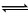[Ag+][Cl]

AgClAg+ + Cl

a-s                    s           s

Ksp = S2

Simple Solubility         Ax ByxAy+ + yB–x

Ksp = (xs)x (ys)y = xx.yy (s)x+y

 Ksp = xx.yy(s)x+y

Factors Affecting Solubility:

• Effect of common ions on solubility

Because of the presence of common ion the solubility of the salt decreases.

AgClAg+      +          Cl

-                       s                       s

Added NaCl, which is having common ion as that of sparingly soluble salt, AgCl.

NaCl    →        Na+      +       Cl

C                     0                    0

-                       C         C

So, added electrolyte, will decrease the solubility of AgCl

AgClAg+ + Cl

S'            S'

Ksp = S' (S' + C)

Assumption: C >>> S'

Hence S' + C »C

Ksp = s1 x C

S1 = ksp / C

• Simultaneous Solubility:

When sparingly soluble salts are added in water simultaneously, there will be two simultaneous equilibria in the solution

AgClAg+      +     Cl

S1+S2          S1                                 Ksp1

AgClAg+      +     Br

S1+S2          S2                                  Ksp2

Ksp1 = (s1 + s2)s1

Ksp2 = (s1 + s2)s2

K1P1+K1P2 = (S1+s2)

From (1)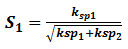• Solubility in presence of hydrolysis:

When the ion from sparingly soluble salt, undergo hydrolysis Hence, we have to follow different procedure to calculate solubility of these salts.

AgCN(s)Ag+ + CN

s1         s-x

CN + H2OHCN + OH

s-x                      x       x

ksp=[Ag+] [CN]                                              … 1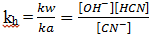… 2

Multiply (1) and (2)

Ksp  x Kh = [Ag+] [HCN]

Since [HCN] = [OH]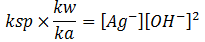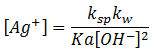Effect of complex formation:

AgCl(s)Ag+ (ag) + Cl (ag)

Ag+ + 2NH3[Ag(NH3)2]+ K= stability constant

S          C

-           C-2S                S

Reverse the Reaction,

[Ag(NH3)2]+Ag+ + 2 NH3

S                           -      C-2S

s-x                   x          C-2s+2x

Since, Kd is very very low,

Hence, c – 2s + 2x ≈ C – 2S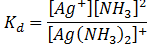Ksp = x × s                                                                   … 1

Put value of Kd in eq. 1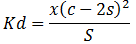S =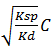Concept of Precipitation: Less the value of S, less the solubility of the salt..Hence, more the extent of precipitation of salt.

AgClAg+ + Cl

With the knowledge of solubility product of a sparingly soluble substance, we can predict whether under certain given conditions that substance would be precipitated or not. It may be remembered that a substance gets precipitated when the ionic product i.e. the product of concentrations of its ions present in a solution exceeds the solubility product of the substance,

Ki = [Ag+] [Cl]

If         Kip>Ksp          Precipitation Occurs

If         Kip<Ksp          Unsaturated Solution

If         kip = Ksp         Saturated Solution

Example : 104 Molar Mg(NO3)2 with pH = 9 and if Ksp (Mg(OH)2) = 8.9 × 1012

1. Will precipitation takes place or not.

2. At what minimum value of pH precipitation will occur.

a­)  Mg(NO3)2     →    Mg2+ + 2 NO3

104                -             -

-                  104          10–4

Mg(OH2)Mg2+ + 20H

Kip = (10–4) (10–5)2

Ki = 10–14

Ksp of Mg(OH)2 = 8.9 × 10–12

Ksp>Kip

Hence solution is unsaturated. No precipitation will occur.

For Mg (OH)2

Ksp = [Mg2+][OH]2

8.9 × 10–12 = 10–4[OH]2

[OH] = 2.9 × 10–4

pOH = 3.83

pH = 10.47

Hence pH should be greater than 10.47 for the precipitation to occur.

Preferential precipitation of an insoluble salt:

Silver chloride is insoluble or sparingly soluble, to be more accurate. So is silver iodide. The question arises as to what would happen if potassium iodide solution is added to silver chloride.

AgCl + KIKCl + AgI

Sparingly                     Sparingly
soluble                         soluble

Would the reaction take place towards the right, i.e. would the precipitated of siler chloride change into the precipitate of silver iodide.

For answer, it is necessary to look their respective solubility products.

Solubility product of silver chloride

Ksp (AgCl) = [Ag+] [Cl] = 1.56 × 10–10

And that of silver iodide

Ksp(AgI) = (Ag+)(I) = 0.94 × 10–16

Example :  0.1 Molar concentration of Cl ion and 103 molar chromate CrO42 ions are present in a solution. If AgNO3 is added which will precipitate first

If         Ksp (AgCl)=1012

Ksp (Ag2CrO4) 1010

2.  What will be the concentration of Cl ions when Ag2CrO4 begins to precipitate. Also find the percentage of Cl left with respect to original.

Solution           AgClAg+ Cl

-             S        S

Ksp = S2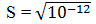S = 10–6

For Ag2CrO4

Ag2CrO42Ag+ + CrO42–

Ksp = (2S)2S

10–10 = 4S3

S = (0.25 × 10–10) 1/3

S = 2.9 × 10–4

Since, solubility of AgClis less than solubility of Ag2CrO4.

Then, obviously AgCl will precipitate first

b)  When Ag2CrO4 begins to precipitate, concentration of CrO42– would be

10–10 = [Ag+]2[CrO42–]

10–10 = [Ag+] × 10–3

[Ag+] = 3.1 × 10–4

For AgCl

Ksp = [Ag+][Cl]

10–12 = 2.9 × 10–4 [Cl]

Cl = 3.4 × 10–9

Percentage of Cl left =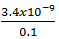Percentage of Cl left = 3.4 × 10–6 %

Offer running on EduRev: Apply code STAYHOME200 to get INR 200 off on our premium plan EduRev Infinity!

## Physical Chemistry

66 videos|94 docs|32 tests

,

,

,

,

,

,

,

,

,

,

,

,

,

,

,

,

,

,

,

,

,

;